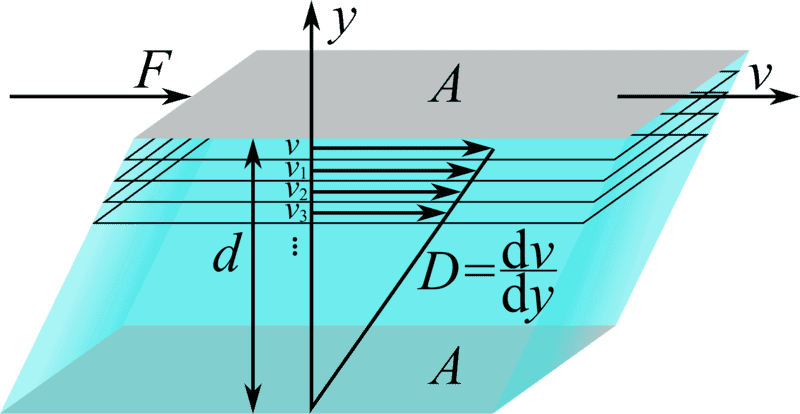# What does 'dv and dx' mean in f = eta X A (dv/dx)?

• Indranil
CHANGEIn summary, the conversation discusses the meaning of "dv" and "dx" in the equation for coefficient of viscosity. The experts explain that "dv" represents the rate of change of velocity with respect to position, and "dx" represents the distance. They also mention that this equation can be written in infinitesimal form as "dv/dx" and give examples of how it is used in different scenarios. f

## Homework Statement

What does 'dv and dx' mean in f = eta A (dv/dx) in coefficient of viscosity?

2. Homework Equations

What does 'dv and dx' mean in f = eta A (dv/dx) in coefficient of viscosity?

## The Attempt at a Solution

As I know v = velocity and x = distance. then what does 'dv' and 'dx' express here. Kindly explain.

Like any derivative, it means the rate at which v varies with respect to x. Imagine running at top speed on asphalt and then you hit a muddy field that gets muddier the farther in you get. Even though you are pumping your muscles as hard as you can, the deeper you get, the lower your speed. The rate at which your speed decreases with distance is dv/dx, in this example a negative number.

Hi Indranil:

I am not sure I understand what is puzzling you. I will tell you what it means to me, and I hope that will be helpful.

dv/dx is the rate of change of velocity as position changes.

You can also write dv/dx = dv/dt / dx/dt.
Do you understand that? Since dx/dt = v, and dv/dt = a (acceleration) you can say that the meaning of dv/dt is the value you get when you divide the acceleration by the velocity.

I hope this helps.

Regards,
Buzz

Here's what Wikipedia has to say about it: https://de.wikipedia.org/wiki/Viskosität (They used ##y## instead of ##x##.)In the experiment, it can be shown that, ideally, the force ##F## necessary to move the top plate is proportional to the area ##A##, the speed difference ##\Delta v## and antiproportional to the spacing of the plates ##\Delta y##, that is:
##F \sim A## and ##F \sim \Delta v## and ##F \sim \dfrac{1}{\Delta y}##

This gives the equation ##F = \eta A {\dfrac{\Delta v}{\Delta y}}## and as continuous, infinitesimal form ##F = \eta A {\dfrac{d v}{d y}}##.

#### Attachments

•Chestermiller and Delta2
I agree with @fresh_42 . This equation is typically used to introduce Newton's law of viscosity, based on the most simple example. In this example, you have a fluid contained between 2 large parallel plates, with the lower plate (y=0) stationary and the upper plate y=h) moving at velocity V in the positive x-direction. The fluid x-velocity in the region between the plates is linear, and given by:$$v_x=V\frac{y}{h}$$. The force in the positive x-direction required to cause the fluid to shear with this velocity profile is given by Newton's law of viscosity as:
$$F=\eta A\frac{dv_x}{dy}=\eta A\frac{V}{h}$$where ##\eta## is the fluid viscosity and A is the plate area. The derivative of the x-velocity with respect to y is referred to as the "shear rate."

The example given by Buzz Bloom, rather than involving shear of the fluid, is referred to as an elongational deformation. In this case, dv/dx is called the "rate of elongation", and the tensile force involves 3 times the viscosity.

•Indranil and Delta2
Delta v\Delta X = Viscosity Gradient

Change in Velocity/Change in Depth = VELOCITY GRADIANT = Uniformly CHANGE

dv/dx = Small CHANGE IN velocity / small change in depth Non Uniform